×#### Thank you for registering.

One of our academic counsellors will contact you within 1 working day.

Click to Chat

1800-1023-196

+91-120-4616500

CART 0

• 0

MY CART (5)

Use Coupon: CART20 and get 20% off on all online Study Material

ITEM
DETAILS
MRP
DISCOUNT
FINAL PRICE
Total Price: Rs.

There are no items in this cart.
Continue Shopping• Complete JEE Main/Advanced Course and Test Series
• OFFERED PRICE: Rs. 15,900
• View Details

```Chapter 9: Arithmetic Progressions Exercise 9.6

Question: 1

Find the sum of the following Arithmetic Progression.

(i) 50, 46, 42,... To 10 terms

(ii) 1, 3, 4, 7, . . . 26 to 12 terms.

(iii) 3, 9/2, 6, 15/2,.... to 25 terms.

(iv) 41, 36, 31, . . .  To 12 terms.

(v) a + b, a - b, a -3b,... To 22 terms.

(vi) (x - y)2 , (x2, y2), (x + y)2, . . . to n terms.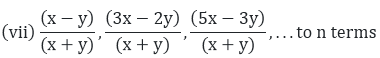(viii) – 26, – 24, – 22, . . . to 36 terms.

Solution:

In the given problem, we need to find the sum of terms for different A.P.

So, here we use the following formula for the sum of n terms of an A.P.,

Sn = n/2[2a + (n − 1)d]

Where; a = first term for the given A.P.

d = common difference of the given A.P.

n = number of terms

(i) 50, 46, 42,... To 10 terms

Common difference of the A.P. (d) = a2 - a1 = 46 - 50 = – 4

Number of terms (n) = 10

First term for the given A.P. (a) = 50 So, using the formula we get,

S10 = 10/2[2(50) + (10 − 1)(−4)] = (5)

[100 + (9)(-4)] = (5)

[100 - 36] = (6)

 = 320

Therefore, the sum of first 10 terms of the given A.P. is 320.

(ii) 1, 3, 4, 7, . . . 26 to 12 terms.

Common difference of the A.P. (d) = a2 - a1 = 3 - 1 = 2

Number of terms (n) = 12 First term (a) = 1 So, using the formula we get,

S12 = 12/2[2(1) + (12 − 1)(2)] = (6)

[2 + (11)(2)] = (6)

[2 + 22 ] = (6)

 = 144

Therefore, the sum of first 10 terms of the given A.P. is 144

(iii) 3, 9/2, 6, 15/2,.... to 25 terms.

Common difference here is (d):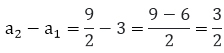Number of terms (n) = 25 First term of the A.P. (a) = 3 So, using the formula we get,

S25 = 25/2[2(3) + (25 − 1)(3/2)]

= (25/2)[6+(72/2)(3/2)]

= (25/2)[6+(72/2)] = (25/2)[6+36]

= (25/2) = (25)(21) = 525

Therefore, the sum of first 12 terms for the given A.P. is 162.

(iv) 41, 36, 31, . . .  to 12 terms.

Common Difference of the A.P. (d) = a2 - a1 = 36 - 41 = -5

Number of terms (n) = 12

First term for the given A.P. (a) = 41 So, using the formula we get,

S12 = 12/2[2(41) + (12 − 1)(−5)]

= (6)[82 + (11)(-5)]

= (6)[82 - 55]

= (6) =162

Therefore, the sum of first 12 terms for the given A.P. 162

(v) a + b, a - b, a -3b,... to 22 terms.

Common difference of the A.P. (d)

= a2 - a1 = (a -  b) - (a + b)

= a - b - a - b = -2b

Number of terms (n) = 22

First term for the given A.P. (a) = a + b So, using the formula we get,

S22 = 22/2[2(a + b) + (22 − 1)( −2b)] = (11)

[2(a + b) + (22 - 1)(-2b)]

= (11)[2a + 2b + (21)(-2b)]

= (11)[2a + 2b - 42b]

= (11)[2a - 40b] = 22a - 40b

Therefore, the sum of first 22 terms for the given A.P. is: 22a - 40b

(vi) (x - y)2 , (x2, y2), (x + y)2, . . . to n terms.

Common difference of the A.P. (d) = a2 - a1 = (x2 - y2) - (x - y)2 = x2 + y2 - (x2 + y2 - 2xy) = 2xy

Number of terms (n) = n

First term for the given A.P. (a) = (x - y)2

So, using the formula, we get.

Sn = n/2[2(x − y)2 + (n − 1)2xy]

Now, taking 2 common from both the terms inside bracket, we get

= n/2(2)[(x − y)2 + (n − 1)xy]

= (n)[(x - y)2 + (n - 1 )xy]

Therefore, the sum of first n terms of the given A.P. is (n)[(x - y)2 + (n - 1)xy]Common difference of the A.P. (d)

= a2 - a1So, using the formula we get,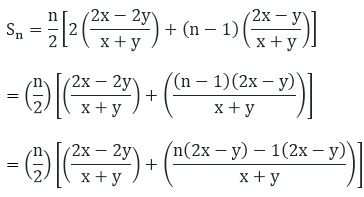On further solving, we getTherefore, the sum of first n terms for the given A.P. is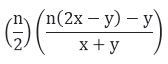(viii) – 26, – 24, – 22, . . . to 36 terms.

Common difference of the A.P. (d)

= a2 - a1 = (-24) - (-26) = -24 + 26 = 2

Number of terms (n) = 36

First term for the given A.P. (a) = -26

So, using the formula we get,

Sn = (36/2)[2(−26) + (36 − 1)(2)] = (18)

[- 52 + (35)(2) = (18)

[- 52 + 70] = (18)

(18) = 324

Therefore, the sum of first 36 terms for the given A.P. is 324

Question: 2

Find the sum to n term of the A.P. 5, 2, –1, –4, –7, . . .

Solution:

In the given problem, we need to find the sum of the n terms of the given A.P. 5, 2, -1,- 4, – 7,....

So, here we use the following formula for the sum of n terms of an A.P.,

Sn = n/2[2a + (n − 1)d]

Where; a = first term for the given A.P. d = common difference of the given A.P. and n = number of terms For the given A.P. (5, 2, -1,- 4,- 7,...),

Common difference of the A.P. (d) = a2- a1 = 2 - 5 = – 3

Number of terms (n) = n

First term for the given A.P. (a) = 5

So, using the formula we get,

Sn = n/2[2(5) + (n − 1)(−3)]

= n/2[10 + (−3n + 3)]

= n/2[10 − 3n +3 ]

= n/2[13 − 3n]

Therefore, the sum of first n terms for the given A.P. is n/2[13 − 3n]

Question: 3

Find the sum of n terms of an A.P. whose nth terms is given by an = 5 - 6n.

Solution:

Here, we are given an AP, whose nth term is given by the following expression, an = 5 - 6n

So, here we can find the sum of the n terms of the given A.P., using the formula,

Sn = (n/2)(a + l)

Where, a = the first term l = the last term So, for the given AP,

The first term (a) will be calculated using n = 1 in the given equation for nth term of A.P.

a = 5 - 6(1) = 5 – 6 = -1

Now, the last term (l) or the nth term is given an = 5 - 6n

So, on substituting the values in the formula for the sum of n terms of an AP.,

we get, S/n =(n/2)[(−1) + 5 − 6n]

= (n/2)[4 − 6n]

= (n/2)(2)[2 − 3n]

= (n)(2 - 3n)

Therefore, the sum of the n terms of the given A.P. is (n) (2 - 3n)

Question: 4

Find the sum of first 15 term of each of the following sequences having nth term as:

(i) an = 3 + 4n

(ii) bn = 5 + 2n

(iii) xn = 6 - n

(iv) yn = 9 – 5n

Solution:

(i) Here, we are given an A.P. whose nth term is given by the following expression,

an = 3 + 4n

We need to find the sum of first 15 term & n ,

So, here we can find the sum of the n terms of the given A.P., using the formula,

Sn = n/2(a + l)

Where, a = the first term l = the last term So, for the given AP,

The first term (a) will be calculated using n = 1 in the given equation for nth term of A.P.

a = 3 + 4(1) = 3 + 4 =7

Now, the last term (l) or the nth term is given l = an = 3 + 4n

So, on substituting the values in the formula for the sum of n terms of an A.P,

we get, Sn = 15/2(7 + 3 + 4(15))

= 15/2(10 + 60)

= 15/2(70)

= (15)(35)

= 525

Therefore, the sum of the 15 terms of the given A.P. is S15 = 525

(ii) Here, we are given an A.P. whose nth term is given by the following expression,

bn = 5 + 2n

We need to find the sum of first 15 term & n,

So, here we can find the sum of the n terms of the given A.P., using the formula,

Sn = n/2(a + l)

Where, a = the first term l = the last term So, for the given AP,

The first term (a) will be calculated using n = 1 in the given equation for nth term of A.P.

b = 5 + 2(1) = 5 + 2 = 7

Now, the last term (l) or the nth term is given l = bn = 5 + 2n

So, on substituting the values in the formula for the sum of n terms of an A.P, we get,

Sn = 15/2(7 + 5 + 2(15))

= 15/2(12 + 30)

= 15/2(42)

= (15)(21)

= 315

Therefore, the sum of the 15th term of the given A.P. is 315

(iii) Here, we are given an A.P. whose nth term is given by the following expression, xn = 6 - n

We need to find the sum of first 15 term & n , So, here we can find the sum of the n terms of the given A.P., using the formula, Sn = n/2(a + l)

Where, a = the first term l = the last term So, for the given AP,

The first term (a) will be calculated using n = 1 in the given equation for nth term of A.P.

b = 6 - 1 = 5

Now, the last term (l) or the nth term is given l = xn = 6 - n

So, on substituting the values in the formula for the sum of n terms of an A.P, we get,

Sn = 15/2((5) + 6 − (15))

= 15/2(11 − 15)

= 15/2(−4)

= (15)(-2)

= -30

Therefore, the sum of the 15 terms of the given A.P. is -30.

(iv) Here, we are given an A.P. whose nth term is given by the following expression, yn = 9 - 5n

We need to find the sum of first 15 term & n,

So, here we can find the sum of the n terms of the given A.P., using the formula, Sn = n/2(a + l)

Where, a = the first term l = the last term So, for the given AP,

The first term (a) will be calculated using n = 1 in the given equation for nth term of A.P.

b = 9 – 5 (1) = 9 - 5 = 4

Now, the last term (l) or the nth term is given l = bn = 9 - 5n

So, on substituting the values in the formula for the sum of n terms of an A.P, we get,

Sn = 15/2((4) + 9 − 5(15))

= 15/2(13 − 75)

= 15/2(−62)

= (15)(-31)

= – 465

Therefore, the sum of the 15 terms of the given A.P. is – 465

Question: 5

Find the sum of first 20 terms the sequence whose nth term is an = An + B.

Solution:

Here, we are given an A.P. whose nth term is given by the following expression an = An + B

We need to find the sum of first 20 terms.

So, here we can find the sum of the n terms of the given A.P., using the formula,

Sn = n/2(a + l)

Where, a = the first term l = the last term So, for the given AP,

The first term (a) will be calculated using n = 1 in the given equation for nth term of A.P.

a = A(1) + B = A + B

Now, the last term (l) or the nth term is given l = an = An + B

So, on substituting the values in the formula for the sum of n terms of an A.P., we get,

S20 = 20/2((A + B) + A(20) + B)

= 10[21A + 2B]

= 210A + 20B

Therefore, the sum of the first 20 terms of the given A.P. is 210 A + 20B

Question:6

Find the sum of first 25 terms of an A.P whose nth term is given by an = 2 - 3n.

Solution:

Here, we are given an A.P. whose nth term is given by the following expression, an = 2 – 3n

We need to find the sum of first 25 terms.

So, here we can find the sum of the n terms of the given AP., using the formula,

Sn = n/2(a + l)

Where, a = the first term l = the last term So, for the given AP,

The first term (a) will be calculated using n= 1 in the given equation for nth term of A.P.

a =2 -3(1) = 2 – 3 = -1

Now, the last term (1) or the nth term is given l = an = 2 - 3n

So, on substituting the values in the formula for the sum of n terms of an AP., we get,

S25 = 25/2[(−1) + 2 − 3(25)]

= 25/2[1 − 75]

= (25)(-37)

= – 925

Therefore, the sum of the 25 terms of the given A.P. is – 925

Question: 7

Find the sum of first 25 terms of an A.P whose nth term is given by an = 7 - 3n.

Solution:

Here, we are given an AP. whose nth term is given by the following expression, an = 7 - 3n.

We need to find the sum of first 25 terms.

So, here we can find the sum of the n terms of the given AP., using the formula, Sn = n/2(a + l)

Where, a = the first term l = the last term So, for the given AP,

The first term (a) will be calculated using n= 1 in the given equation for nth term of A.P.

a = 7 - 3 = 4

Now, the last term (l) or the nth term is given l = an = 7 - 3n

So, on substituting the values in the formula for the sum of n terms of an AP., we get,

Sn = 25/2[(4) + 7 − 3(25)]

= 25/2[11 − 75]

= 25/2[−64]

= (25)(−32)

= −800

Therefore, the sum of the 25 terms of the given A.P. is S­n = – 800

Question: 8

If the sum of a certain number of terms starting from first term of an A.P. is 25, 22, 19, . . ., is 116. Find the last term.

Solution:

In the given problem, we have the sum of the certain number of terms of an A.P. and we need to find the last term for that A.P.

So here, let us first find the number of terms whose sum is 116.

For that, we will use the formula, Sn = n/2[2a + (n − 1)d]

Where; a = first term for the given A.P. d = common difference of the given A.P. n = number of terms So for the given A.P.(25, 22, 19,...)

The first term (a) = 25

The sum of n terms Sn = 116

Common difference of the A.P.

(d) = a2 - a1

= 22 - 25

= -3

So, on substituting the values in the formula for the sum of n terms of an A.P., we get,

⟹ 116 = n/2[2(25) + (n − 1)(−3)]

⟹ (n/2)[50 + (−3 + 3)]

⟹ (n/2)[53 − 3n]

⟹ 116 × 2

= 53n - 3n2

So, we get the following quadratic equation,

3n2 - 53n + 232 = 0

On solving by splitting middle term, we get,

⟹ 3n2 - 24n - 29n + 232 = 0

⟹ 3n( n - 8 ) - 29 ( n - 8 ) = 0

⟹ (3n - 29)( n - 8 ) = 0 Further, 3n - 29 = 0

⟹ n = 293 Also, n - 8 = 0

⟹ n = 8

Since, n cannot be a fraction, so the number of terms is 8. So, the term is:

a8 = a1 + 7d = 25 + 7(-3) = 25 - 21 = 4

Therefore, the last term of the given A.P. such that the sum of the terms is 116 is 4.

Question: 9

(i) How many terms of the sequence 18, 16, 14....  should be taken so that their sum is 0 (Zero).

(ii) How many terms are there in the A.P. whose first and fifth terms are -14 and 2 respectively and the sum of the terms is 40?

(iii) How many terms of the A.P. 9, 17, 25, . . . must be taken so that their sum is 636?

(iv) How many terms of the A.P. 63, 60, 57, . . . must be taken so that their sum is 693?

(v) How many terms of the A.P. is 27, 24, 21. . . should be taken that their sum is zero?

Solution:

(i) AP. is 18, 16,14,... So here, let us find the number of terms whose sum is 0.

For that, we will use the formula,

Sn = n/2[2a + (n − 1)d]

where; a = first term for the given A.P. d = common difference of the given A.P. n = number of terms The first term (a) = 18 The sum of n terms (Sn) = 0 Common difference of the A.P.

(d) = a2  - a1 = 16 - 18 = – 2

So, on substituting the values in the formula for the sum of n terms of an AP., we get

⟹ 0 = n/2[2(18) + (n − 1)(−2)]

⟹ 0 = n/2[36 + (−2n + 2)]

⟹ 0 = n/2[38 − 2n] Further, n/2

⟹ n = 0 Or, 38 - 2n = 0

⟹ 2n = 38

⟹ n = 19

Since, the number of terms cannot be zero; the number of terms (n) is 19

(ii) Here, let us take the common difference as d. So, we are given, First term (a1) = -14 Filth term (a5) = 2 Sum of terms (Sn) = 40

Now, a5 = a1 + 4d

⟹ 2 = -14 + 4d

⟹ 2 + 14 = 4d

⟹ 4d = 16

⟹ d = 4

Further, let us find the number of terms whose sum is 40.

For that, we will use the formula,

Sn = n/2[2a + (n − 1)d]

Where; a = first term for the given A.P. d = common difference of the given A.P. n = number of terms

The first term (a1) = -14

The sum of n terms (Sn) = 40

Common difference of the A.P. (d) = 4

So, on substituting the values in the formula for the sum of n terms of an A.P., we get,

⟹ 40 = n/2[2(−14) + (n − 1)(4)]

⟹ 40 = n/2[−28 + (4n − 4)]

⟹ 40 = n/2[−32 + 4n]

⟹ 40(2) = - 32n + 4n2

So, we get the following quadratic equation,

4n2 - 32n - 80 = 0

⟹ n2 - 8n + 20 = 0

On solving by splitting the middle term, we get

4n2 - 10n + 2n + 20 = 0

⟹ n(n - 10) + 2( n - 10 ) = 0

⟹ (n + 2)(n - 10) = 0 Further, n + 2 = 0

⟹ n = -2 Or, n - 10 = 0

⟹ n = 10

Since the number of terms cannot be negative.

Therefore, the number of terms (n) is 10.

(iii) AP is 9, 17, 25,...

So here, let us find the number of terms whose sum is 636.

For that, we will use the formula,

Sn = n/2[2a + (n − 1)d]

Where; a = first term for the given A.P. d = common difference of the given A.P. n = number of terms

The first term (a) = 9 The sum of n terms (Sn) = 636

Common difference of the A.P.

(d) = a2  - a1 = 17 - 9 = 8

So, on substituting the values in the formula for the sum of n terms of an AP., we get,

⟹ 636 = n/2[2(9) + (n − 1)(8)]

⟹ 636 = n/2[18 + (8n − 8)]

⟹ 636(2) = (n)[10 + 8n]

⟹ 1271 = 10n + 8n2

So, we get the following quadratic equation,

⟹ 8n2 + 10n - 1272 = 0

⟹ 4n2+ 5n - 636 = 0

On solving by splitting the middle term, we get,

⟹ 4n2 - 48n + 53n - 636 = 0

⟹ 4n(n - 12) - 53(n - 12) = 0

⟹ (4n - 53)(n - 12) = 0 Further, 4n - 53 = 0 ⟹ n = 534 Or, n - 12 = 0 ⟹ n = 12

Since, the number of terms cannot be a fraction.

Therefore, the number of terms (n) is 12.

(iv) A.P. is 63, 60, 57,...

So here. let us find the number of terms whose sum is 693.

For that, we will use the formula.

Sn = n/2[2a + (n − 1)d]

Where; a = first term for the given A.P. d = common difference of the given A.P. n = number of terms

The first term (a) = 63

The sum of n terms (Sn) = 693

Common difference of the A.P.

(d) = a2 - a1 = 60 - 63 = –3

So, on substituting the values in the formula for the sum of n terms of an AP we get.

⟹ 693 = n/2[2(63) + (n − 1)(−3)]

⟹ 693 = n/2[163+(−3n + 3)]

⟹ 693 = n/2[129 − 3n]

⟹ 693(2) = 129n - 3n2

So. we get the following quadratic equation.

⟹ 3n2 - 129n + 1386 = 0

⟹ n2 - 43n + 462

On solving by splitting the middle term, we get.

⟹ n2 - 22n - 21n + 462 = 0

⟹ n(n - 22) -21(n - 22) = 0

⟹ (n - 22) (n - 21) = 0 Further, n - 22 = 0 ⟹ n = 22 Or,  n - 21 = 0

⟹ n = 21 Here, 22nd term will be a22 = a1 + 21d = 63 + 21( -3 ) = 63 - 63 = 0

So, the sum of 22 as well as 21 terms is 693.

Therefore, the number of terms (n) is 21 or 22
(v) A.P. is 27, 24, 21. . .

So here. let us find the number of terms whose sum is 0. For that, we will use the formula.

Sn = n/2[2a + (n − 1)d]

Where; a = first term for the given A.P. d = common difference of the given A.P. n = number of terms

The first term (a) = 27

The sum of n terms (Sn) = 0

Common difference of the A.P.

(d) = a2 - a1 = 24 - 27 = -3

So, on substituting the values in the formula for the sum of n terms of an AP we get.

⟹ 0 = n/2[2(27) + (n − 1)( − 3)]

⟹ 0 = (n)[54 + (n - 1)(-3)]

⟹ 0 = (n)[54 - 3n + 3]

⟹ 0 = n [57 - 3n] Further we have, n = 0 Or, 57 - 3n = 0

⟹ 3n = 57

⟹ n = 19

The number of terms cannot be zero,

Therefore, the numbers of terms (n) is 19.

Question: 10

Find the sum of the first

(i) 11 terms of the A.P. : 2, 6, 10, 14,  . . .

(ii) 13 terms of the A.P. : -6, 0, 6, 12, . . .

(iii) 51 terms of the A.P. : whose second term is 2 and fourth term is 8.

Solution:

In the given problem, we need to find the sum of terms for different arithmetic progressions.

So, here we use the following formula for the sum of n terms of an A.P.,

Sn = n/2[2a + (n − 1)d]

Where; a = first term for the given A.P. d = common difference of the given A.P. n = number of terms

(i) 2, 6, 10, 14,... to 11 terms.

Common difference of the A.P.

(d) = a2 - a1 = 10 - 6 = 4

Number of terms (n) = 11

First term for the given A.P. (a) = 2

So, using the formula we get, S11 = 11/2[2(2) + (11 − 1)4]

= 11/2[2(2) + (10)4]

= 11/2[4 + 40]

= 11 × 22

= 242

Therefore, the sum of first 11 terms for the given A.P. is 242

(ii) – 6, 0, 6, 12, ... to 13 terms.

Common difference of the AR

(d) = a2 - a­­1 = 6 - 0 = 6

Number of terms (n) = 13

First term for the given AP (a) = -6

So, using the formula we get,

S13 = 13/2[2(− 6) + (13 –1)6]

= 13/2[(−12) + (12)6]

= 13/2 = 390

Therefore, the sum of first 13 terms for the given AR is 390

(iii) 51 terms of an AP whose a2 = 2 and a­­4 = 8

Now, a2 = a + d

2 = a + d  ...(i)

Also, a4 = a + 3

8 = a + 3d  ... (2)

Subtracting (1) from (2), we get

2d = 6

d = 3

Substituting d = 3 in (i), we get 2 = a + 3

⟹ a = -1

Number of terms

(n) = 51

First terms for the given A.P.(a) = -1

So, using the formula, we get

Sn = 51/2[2(−1) + (51 − 1)(3)]

= 51/2[−2 + 150]

= 51/2

= 3774

Therefore, the sum of first 51 terms for the A.P. is 3774.

Question: 11

Find the sum of (i) First 15 multiples of 8 (ii) the first 40 positive integers divisible by (a) 3 (b) 5 (c) 6. (iii) all 3 - digit natural numbers which are divisible by 13.

Solution:

In the given problem, we need to find the sum of terms for different arithmetic progressions.

So, here we use the following formula for the sum of n terms of an A.P.,

Sn = n/2[2a + (n − 1)d]

Where: a = first term for the given A.P. d = common difference of the given A.P. n = number of terms
(i) First 15 multiples of 8.

So, we know that the first multiple of 8 is 8 and the last multiple of 8 is 120.

Also, all these terms will form an A.P. with the common difference of 8. So here,

First term (a) = 8 Number of terms (n) = 15

Common difference (d) = 8

Now, using the formula for the sum of n terms, we getTherefore, the sum of the first 15 multiples of 8 is 960 (ii)(a)

First 40 positive integers divisible by 3 So, we know that the first multiple of 3 is 3 and the last multiple of 3 is 120.

Also, all these terms will form an A.P. with the common difference of 3.

So here, First term (a) = 3

Number of terms (n) = 40

Common difference (d) = 3

Now, using the formula for the sum of n terms, we get

Sn = 40/2[2(3) + (40 − 1)3]

= 20[6 + (39)3]

= 20(6 + 117)

= 20(123) = 2460

Therefore, the sum of first 40 multiples of 3 is 2460 (b)

First 40 positive integers divisible by 5 So, we know that the first multiple of 5 is 5 and the last multiple of 5 is 200.

Also, all these terms will form an A.P. with the common difference of 5.

So here, First term (a) = 5

Number of terms (n) = 40

Common difference (d) = 5

Now, using the formula for the sum of n terms, we get

Sn = 40/2[2(5) + (40 − 1)5]

= 20[10 + (39)5]

= 20 (10 + 195)

= 20 (205) = 4100

Therefore, the sum of first 40 multiples of 5 is 4100 (c)

First 40 positive integers divisible by 6 So, we know that the first multiple of 6 is 6 and the last multiple of 6 is 240.

Also, all these terms will form an A.P. with the common difference of 6.

So here, First term (a) = 6

Number of terms (n) = 40

Common difference (d) = 6

Now, using the formula for the sum of n terms, we get

Sn = 40/2[2(6) + (40 − 1)6]

= 20[12 + (39)6]

=20(12 + 234)

= 20(246) = 4920

Therefore, the sum of first 40 multiples of 6 is 4920

(ii) All 3 digit natural number which are divisible by 13 So, we know that the first 3 digit multiple of 13 is 104 and the last 3 digit multiple of 13 is 988.

Also, all these terms will form an AR with the common difference of 13.

So here, First term (a) = 104 Last term (l) = 988

Common difference (d) = 13

So, here the first step is to find the total number of terms.

Let us take the number of terms as n. Now, as we know, an = a + (n − 1)d

So, for the last term,

988 = 104 + (n - 1)13

⟹ 988 = 104 + 13n -13

⟹ 988 = 91 + 13n

⟹ 13n = 897

⟹ n = 69

Now, using the formula for the sum of n terms, we get

Sn = 69/2[2(104) + (69 − 1)13]

= 69/2[208 + 884]

= 69/2

= 69(546)

= 37674

Therefore, the sum of all 3 digit multiples of 13 is 37674

Question: 12

Find the sum:

(i) 2 + 4 + 6 + . . . + 200

(ii) 3 + 11 + 19 + . . . + 803

(iii) (-5) + (-8) + (-11) + . . . + (- 230)

(iv) 1 + 3 + 5 + 7 + . . . + 199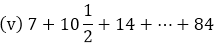(vi) 34 + 32 + 30 + . . . + 10

(vii) 25 + 28 + 31 + . . . + 100

Solution:

In the given problem, we need to find the sum of terms for different arithmetic progressions.

So, here we use the following formula for the sum of n terms of an A.P.,

Sn = n/2[2a + (n - 1)d] or Sn = n/2[a + l]

Where; a = first term of the given A.P. d = common difference of the given A.P. l = last term n = number of terms

(i) 2 + 4 + 6 + ... + 200

Common difference of the A.P.

(d) = a2 - a1 = 6 - 4 = 2

So here, First term (a) = 2

Last term (l) = 200

Common difference (d) = 2

So, here the first step is to find the total number of terms.

Let us take the number of terms as n. Now, as we know, an = a + (n - 1)d

So, for the last term,

200 = 2 + (n - 1)2

200 = 2 + 2n - 2

200 = 2n

Further simplifying, n = 100

Now using the formula for sum of n terms,

S100 = 100/2[a + l] = 50

[2 + 200] = 50 X 202 = 10100

Therefore, the sum of the A.P is 10100

(ii) 3 + 11 + 19 + . . . + 803

Common difference of the A.P.

(d) = a2 - a1 =19 - 11 = 8

So here, First term (a) = 3 Last term (l) = 803

Common difference (d) = 8

So, here the first step is to find the total number of terms.

Let us take the number of terms as n.

Now, as we know, an = a + (n - 1)d

So, for the last term, further simplifying,

803 = 3 + (n - 1)8

⟹ 803 = 3 + 8n - 8

⟹ 803 + 5 = 8n

⟹ 808 = 8n

⟹ n = 101

Now, using the formula for the sum of n terms, we get

S101 = 101/2[a + l]

= 101/2[3 + 803]

= 101/2

= 101(403)

= 40703

Therefore, the sum of the A.P. is 40703

(iii) (-5) + (-8) + (-11) + . . . + (-230)

Common difference of the A.P.

(d) = a2 - a2 = -8 - (-5) = -8 + 5 = -3

So here, First term (a) = -5 Last term (l) = -230

Common difference (d) = -3

So, here the first step is to find the total number of terms.

Let us take the number of terms as n.

Now, as we know, an = a + (n - 1)d

So, for the last term,

-230 = -5 + (n - 1)(-3)

⟹ -230 = -5 -3n +3

⟹ -230 + 2 = -3n

⟹ -228 = -3n

⟹ n = 76

Now, using the formula for the sum of n terms, we get

S76 = 76/2[a + l] = 38[(- 5) + (-230)] = 38(-235) = – 8930

Therefore, the sum of the A.P. is – 8930

(iv) 1 + 3 + 5 + 7 + . . . + 199

Common difference of the A.P.

(d) = a2 - a1 = 3 - 1 = 2

So here, First term (a) = 1 Last term (l) = 199

Common difference (d) = 2 So, here the first step is to find the total number of terms.

Let us take the number of terms as n.

Now, as we know, an = a + (n - 1)d

So, for the last term, 199 = 1 + (n - 1)2

⟹ 199 = 1 + 2n - 2

⟹ 199 + 1 = 2n

⟹ n = 100

Now, using the formula for the sum of n terms, we get

S100 = 100/2[a + l] = 50

[1 + 199] = 50

(200) = 10000

Therefore, the sum of the A.P. is 10000Common difference of the A.P. (d) = a2 - a1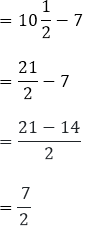So here, First term (a) = 7 Last term (l) = 184

Common difference (d) = 72

So, here the first step is to find the total number of terms.

Let us take the number of terms as n.

Now, as we know, an = a + (n - 1)d

So, for the last term,

84 = 7 + (n − 1) 7/2

84 = 7 + 7n/2 − 7/2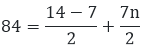84(2) = 7 + 7n

7n = 161

n = 23

Now, using the formula for sum of n terms, we get

Sn = 23/2[2(7) + (23 − 1)7/2]

= 23/2[14 + (22)7/2]

= 23/2[14 + 77]

= 23/2

= 2093/2

Therefore, the sum of the A.P. is 2093/2

(vi) 34 + 32 + 30 + . . . + 10

Common difference of the A.P.

(d) = a2 - a1 = 32 -34 = -2

So here, First term (a) = 34

Last term (l) = 10

Common difference (d) = -2

So, here the first step is to find the total number of terms.

Let us take the number of terms as n. Now, as we know, an = a +(n - 1)d

So, for the last term,

⟹ 10 = 34 + (n - 1)(-2)

⟹ 10 = 34 - 2n + 2

⟹ 10 = 36 - 2n

⟹ 10 - 36 = -2n

Further solving for n,

⟹ -2n = -26

⟹ n = 13

Now, using the formula for the sum of n terms, we get

Sn = 13/2[a + l]

= 13/2[34 + 10]

= 13/2

= 12(22) = 286

Therefore, the sum of the A.P. is 286

(vii) 25 + 28 + 31 + . . . + 100

Common difference of the A.P.

(d) = a2 - a1 = 28 - 25 = 3

So here, First term (a) = 25 Last term (l) = 100

Common difference (d) = 3

So, here the first step is to find the total number of terms.

Let us take the number of terms as n.

Now, as we know, an = a + (n - 1)d

So, for the last term,

100 = 25 + (n - 1)(3)

100 = 25 + 3n - 3

100 = 22 + 3n

100 - 22 = 3n

Further solving for n, 78 = 3n n = 26

Now, using the formula for the sum of n terms, we get

Sn = 26/2[a + l] = 13

[25 + 100] = 13

(125) = 1625

Therefore, the sum of the given A.P. is 1625

Question: 13

The first and the last terms of an A.P. are 17 and 350 respectively. If the common difference is 9, how many terms are there and what is their sum?

Solution:

In the given problem, we have the first and the last term of an A.P. along with the common difference of the AP Here, we need to find the number of terms of the AP and the sum of all the terms.

Here, The first term of the A.P (a) = 17

The last term of the A.P (l) = 350

The common difference of the A.P. = 9

Let the number of terms be n. So, as we know that, l = a + (n - 1)d we get, 350 = 17 + (n- 1) 9

⟹ 350 = 17 + 9n - 9

⟹ 350 = 8 + 9n

⟹ 350 - 8 = 9n

Further solving this, n = 38

Using the above values in the formula,

Sn = n/2[a + l]

⟹ 38/2(17 + 350)

⟹ 19 × 367

⟹ 6973

Therefore, the number of terms is (n) 38 and the sum (Sn) is 6973

Question: 14

The third term of an A.P. is 7 and the seventh term exceeds three times the third term by 2. Find the first term, the common difference and the sum of first 20 terms.

Solution:

In the given problem, let us take the first term as a and the common difference as d. Here, we are given that,

a3 = 7 .... (1)

a7 = 3a3 + 2   .... (2)

So, using (1) in (2), we get,

a7 = 3(7) + 2

= 21 + 2 = 23  .... (3)

Also, we know,

an = a +(n - 1)d

For the 3th term (n = 3), a3 = a + (3 - 1)d

⟹ 7 = a + 2d   (Using 1)

⟹ a = 7 - 2d     .... (4)

Similarly, for the 7th term (n = 7), a7 = a + (7 - I) d 24 = a + 6d   (Using 3)

a = 24 - 6d  .... (5)

Subtracting (4) from (5), we get,

a - a = (23 - 6d) - (7 - 2d)

⟹ 0 = 23 - 6d - 7 + 2d

⟹ 0 = 16 - 4d

⟹ 4d = 16

⟹ d = 4

Now, to find a, we substitute the value of d in  (4), a =7 - 2(4)

⟹ a = 7 - 8

a = -1

So, for the given A.P, we have d = 4 and a = -1

So, to find the sum of first 20 terms of this A.P., we use the following formula for the sum of n terms of an AP,

Sn = n/2[2a + (n − 1)d]

Where; a = first term for the given A.P. d= common difference of the given A.P. n= number of terms So, using the formula for n = 20, we get,

S20 = 20/2[2(−1) + (20 − 1)(4)]

= (10)[-2 + (19)(4)]

= (10)[-2 + 76]

= (10) = 740

Therefore, the sum of first 20 terms for the given A.P. is S20 = 740

Question: 15

The first term of an A.P. is 2 and the last term is 50. The sum of all these terms is 442. Find the common difference.

Solution:

In the given problem, we have the first and the last term of an A.P. along with the sum of all the terms of A.P. Here, we need to find the common difference of the A.P.

Here, The first term of the A.P (a) = 2

The last term of the A.P (I) = 50

Sum of all the terms S„ = 442

Let the common difference of the A.P. be d.

So, let us first find the number of the terms (n) using the formula,

442 = (n/2)(2 + 50)

⟹ 442 = (n/2)(52)

⟹ 26n = 442

⟹ n = 17

Now, to find the common difference of the A.P. we use the following formula, l = a + (n - 1)d

We get, 50 = 2 + (17 - 1)d

⟹ 50 = 2 + 16d

⟹ 16d = 48

⟹ d = 3

Therefore, the common difference of the A.P. is d = 3

Question: 16

If 12th term of an A.P. is -13 and the sum of the first four terms is 24, what is the sum of first 10 terms?

Solution:

In the given problem, we need to find the sum of first 10 terms of an A.P.

Let us take the first term a and the common difference as d Here, we are given that,

a12 = -13 S4 = 24

Also, we know,

an = a + (n - 1)d

For the 12th term (n = 12)

a12 = a + (12 - 1)d - 13

= a + 11d

a = -13 - 11d  .... (1)

So, as we know the formula for the sum of n terms of an A.P. is given by,

Sn = n/2[2a + (n − 1)d]

Where; a = first term for the given A.P. d = common difference of the given A.P. n = number of terms

So, using the formula for n = 4, we get,

S4 = 4/2[2(a) + (4 − 1)d]

⟹ 24 = (2)[2a + (3)(d)]

⟹ 24 = 4a + 6d

⟹ 4a = 24 - 6d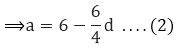Subtracting (1) from (2), we get.On further simplifying for d, we get,⟹ -19 × 2 = 19d

⟹ d = – 2

Now, we have to substitute the value of d in (1),

a = -13 - 11(-2)

a = -13 + 22

a = 9

Now, using the formula for the sum of n terms of an A.P., for n = 10 we have,

S10 = 10/2[2(9) + (10 − 1)(−2)]

= (5)[19 + (9)(-2)]

= (5)(18 - 18) = 0

Therefore, the sum of first 10 terms for the given A.P. is S10 = 0.

Question: 17

Find the sum of first 22 terms of an A.P. in which d = 22 and a22 = 149.

Solution:

In the given problem, we need to find the sum of first 22 terms of an A.P.

Let us take the first term as a. Here, we are given that,

a22 = 149 .... (1)

d = 22 .... (2)

Also, we know,

an = a + ( n - 1) d

For the 22nd term (n = 22),

a22 = a + (22 - 1)d

149 = a + (21) (22)  (Using 1 and 2)

a = 149 - 462

a = - 313 .... (3)

So, as we know the formula for the sum of n terms of an A.P. is given by,

Sn = n/2[2a + (n − 1)d]

where; a = first term for the given A.P. d = common difference of the given A.P. n = number of terms

So, using the formula for n = 22, we get,

S22 = 22/2[2(−313) + (22 − 1)(22)]

= (11)[ – 626 + 462]

= (11)[–164] = – 1804

Therefore, The sum of first 22 terms for the given A.P. is S22 = -1804

Question: 18

In an A.P., if the first tern is 22, the common difference is – 4 and the sum to n terms is 64, find n.

Solution:

In the given problem, we need to find the number of terms of an A.P.

Let us take the number of terms as n. Here, we are given that, a = 22 d = – 4 S„ = 64

So, as we know the formula for the sum of n terms of an A.P. is given by,

Sn = n/2[2a + (n − 1)d]

Where; a = first term for the given A.P. d = common difference of the given A.P. n = number of terms

So, using the formula we get,

⟹ Sn = n/2[2(22) + (n − 1)(−4)]

⟹ 64 = n/2[2(22) + (n − 1)(−4)]

⟹ 64(2) = n(48 - 4n)

⟹ 128 = 48n - 4n2

Further rearranging the terms, we get a quadratic equation,

4n2 - 48n + 128 = 0

On taking 4 common, we get,

n2 - 12n + 32 = 0

Further, on solving the equation for n by splitting the middle term, we get,

n2 - 12n + 32 = 0

n2 - 8n - 4n + 32 = 0

n ( n - 8 ) - 4 ( n - 8 ) = 0

(n - 8) (n - 4) = 0

So, we get n - 8 = 0

⟹ n = 8

Also, n - 4 = 0

⟹ n = 4

Therefore, n = 4 or 8.

Question: 19

In an A.P., if the 5th and 12th terms are 30 and 65 respectively, what is the sum of first 20 terms?

Solution:

In the given problem, let us take the first term as a and the common difference d Here, we are given that,

a5 = 30  .... (1)

a12 = 65 .... (2)

Also, we know,

an = a + (n - 1)d

For the 5th term (n = 5),

a5 = a + (5 - 1)d

30 = a + 4d (Using 1)

a = 30 - 4d   .... (3)

Similarly, for the 12th term (n = 12),

a12 = a + (12 - 1) d

65 = a + 11d (Using 2)

a = 65 - 11d .... (4)

Subtracting (3) from (4), we get,

a - a = (65 - 11d) - (30 - 4d)

0 = 65 - 11d - 30 + 4d

0 = 35 - 7d

7d = 35

d = 5

Now, to find a, we substitute the value of d in (4).

a = 30 - 4(5)

a = 30 - 20

a = 10

So, for the given A.P.

d = 5 and a = 10

So, to find the sum of first 20 terms of this A.P., we use the following formula for the sum of n terms of an A.P.,

Sn = n/2[2a + (n − 1)d]

Where;

a = first term of the given A.P.

d = common difference of the given A.P.

n = number of terms

So, using the formula for n = 20, we get

S20 = 20/2[2(10) + (20 − 1)(5)]

= (10)[20 + (19)(5)]

= (10)[20 + 95]

= (10)

= 1150

Therefore, the sum of first 20 terms for the given A.P. is 1150

Question: 20

Find the sum of first 51 terms of an A.P. whose second and third terms are 14 and 18 respectively.

Solution:

In the given problem, let us take the first term as a and the common difference as d. Here, we are given that,

a2 = 14 .... (1)

a3 = 18 .... (2)

Also, we know,

an = a + (n - 1)d

For the 2nd term (n = 2),

⟹ a2 = a + (2 - 1)d

⟹ 14 = a + d (Using 1)

⟹ a = 14 - d .... (3)

Similarly, for the 3rd term (n = 3),

⟹ a3 = a + (3 - 1)d

⟹ 18 = a + 2d (Using 2)

⟹ a = 18 - 2d .... (4)

Subtracting (3) from (4), we get,

a - a = (18 - 2d) - (14 - d)

0 = 18 - 2d - 14 + d

0 = 4 - d

d = 4

Now, to find a, we substitute the value of d in (4),

a = 14 - 4

a = 10

So, for the given A.P.

d = 4 and a = 10

So, to find the sum of first 51 terms of this A.P., we use the following formula for the sum of  n terms of an A.P.,

Sn = n/2(2a + (n − 1)d)

Where,

a = the first term of the A.P.

d = common difference of the A.P.

n = number of terms So, using the formula for

n = 51,

we get

S51 = 51/2[2(10) + (51 - 1)(4)]

= 51/2[20 + (40)4]

= 51/2

= 51(110)

= 5610

Therefore, the sum of the first 51 terms of the given A.P. is 5610

Question: 21

The first term of an A.P. is 5, the last term is 45 and the sum is 400. Find the number of terms and the common difference.

Solution:

Let a be the first term and d be the common difference. We know that, sum of first n terms is

Sn = n/2(2a + (n − 1)d)

Where, a = the first term of the A.P.

d = common difference of the A.P.

n = number of terms Also, nth term = an = a + (n - 1)

According to the question, First term (a) = 5, last term (an) = 45 and sum of n terms (Sn) = 400

Now,

an = a + (n - 1)d

⟹ 45 = 5 + (n - 1)d

⟹ 40 = nd - d

⟹ nd - d = 40 .... (1)

Also,

Sn = n/2(2(a) + (n − 1)d)

400 = n/2(2(5) + (n − 1)d)

800 = n (10 + nd - d)

800 = n (10 + 40) from (1)

n = 16 .... (2)

On substituting (2) in (1), we get

nd - d = 40

16d - d = 40

15d = 40

d = 8/3

Thus, common difference of the given A.P. is 83.

Question: 22

In an A.P. the first term is 8, nth term is 33 and the sum of first n term is 123. Find n and the d, the common difference.

Solution:

In the given problem, we have the first and the nth term of an A.P. along with the sum of the n terms of A.P.

Here, we need to find the number of terms and the common difference of the A.P

Here, The first term of the A.P (a) = 8

The nth term of the A.P (l)= 33 Sum of all the terms Sn = 123

Let the common difference of the A.P. be d.

So, let us first find the number of the terms (n) using the formula,

123 = (n/2)(8 + 33)

123 = (n/2)(41)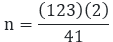n = 246/41

n = 6

Now, to find the common difference of the A.P. we use the following formula,

l = a + (n - 1)d we get

33 = 8 + (6 - 1)d

33 = 8 + 5d

5d = 25

d = 5

Therefore, the number of terms is n = 6 and the common difference of the A.P. is

d = 5.

Question: 23

In an A.P. the first term is 22, nth term is -11 and the sum of first n term is 66. Find n and the d, the common difference.

Solution:

In the given problem, we have the first and the nth term of an A.P. along with the sum of the n terms of A.P.

Here, we need to find the number of terms and the common difference of the A.P.

Here, The first term of the A.P (a) = 22

The nth term of the A.P (l) = -11

Sum of all the terms S = 66

Let the common difference of the A.P. be d.

So, let us first find the number of the terms (n) using the formula,

66 = (n/2)[22 + (−11)]

66 = (n/2)[22 − 11]

(66)(2) = n(11)

6 × 2 = n

n = 12

Now, to find the common difference of the A.P. we use the following formula,

l = a + (n - 1)d we get,

- 11 = 22 + (12 - 1 )d -11 = 22 + 11d

11d = – 33

d = – 3

Therefore, the number of terms is n = 12 and the common difference d = -3

Question: 24

The first and the last terms of an A.P. are 7 and 49 respectively. If sum of all its terms is 420, find the common difference.

Solution:

Let a be the first term and d be the common difference.

We know that, sum of first n terms is:

Sn = n/2(2a + (n − 1)d)

Also, nth term (an) = a + (n - 1)d

According to question, first term (a) = 7 last term (an) = 49 and sum of n terms (Sn) = 420

Now,

an = a + (n - 1)d

⟹ 49 = 7 + (n - 1)d

⟹ 43 = nd - d

⟹ nd - d = 42  ..... (1)

Also,

Sn = n/2(2(7) + (n − 1)d)

⟹ 840 = n[14 + nd - d]

⟹ 840 = n[14 + 42] [from (1)]

⟹ 840 = 54n

⟹ n = 15 .... (2)

on substituting (2) in (1), we get

nd - d = 42

⟹ 15d - d = 42

⟹ 14d = 42

⟹ d = 3

Thus, the common difference of the given A.P. is 3.

Question: 25

The sum of first q terms of an A.P. is 162. The ratio of its 6th term to its 13th term is 1: 2. Find the first and 15th term of the A.P.

Solution:

Let a be the first term and d be the common difference. We know that, sum of first n terms is:

Sn = n/2(2a + (n − 1)d)

Also, nth term = an = a + (n - 1)d

According to the question,

Sq = 162 and a6 : a13 = 1 : 2

Now,

2a6 = a13

⟹ 2 [a + (6 - 1d)] = a + (13 - 1)d

⟹ 2a + 10d = a + 12d

⟹ a = 2d  .... (1)

Also, S9­ = 162

⟹ S9 = 9/2(2a + (9 − 1)d)

⟹ 162 = 9/2(2a + 8d)

⟹ 162 × 2 = 9[4d + 8d] [from (1)]

⟹ 324 = 9 × 12d

⟹ d = 3

⟹ a = 2d [from (1)]

⟹ a = 6

Thus, the first term of the A.P. is 6

Now, a15 = a + 14d = 6 + 14 × 3 = 6 + 42

a 15 = 48

Therefore, 15th term of the A.P. is 48

Question: 26

If the 10th term of an A.P. is 21 and the sum of its first 10 terms is 120, find its nth term.

Solution:

Let a be the first term and d be the common difference.

We know that, sum of first n terms is:

Sn = n/2(2a + (n − 1)d) and nth term is given by:

an = a + (n - 1)d

Now, given in question,

S10 = 120

⟹ 120 = 10/2(2a + (10 − 1)d)

⟹ 120 = 5(2a + 9d)

⟹ 24 = 2a + 9d .... (1)

Also, a10 = 21

⟹ 21 = a + (10 - 1)d

⟹ 21 = a + 9d .... (2)

Subtracting (2) from (1), we get

24 - 21 = 2a + 9d - a - 9d

a = 3 Putting a = 3 in equation (2), we have

3 + 9d = 21

9d = 18

d = 2

So, we have now first term = 3 common difference = 2

Therefore, the nth term can be calculated by:

an = a + (n - 1)d = 3 + (n - 1)2

= 3 + 2n -2

= 2n + 1

Therefore, the nth term of the A.P is (an) = 2n + 1

Question: 27

The sum of first 7 terms of an A.P. is 63 and the sum of its next 7 terms is 161. Find the 28th term of this A.P.

Solution:

Let a be the first term and d be the common difference.

We know that, sum of first n terms

Sn = n/2(2a + (n − 1)d)

It is given that sum of the first 7 terms of an A.P. is 63.

And sum of next 7 terms is 161. Sum of first 14 terms = Sum of first 7 terms + sum of next 7 terms

= 63 + 161 = 224

Now,

S7 = 7/2(2a + (7 − 1)d)

⟹ 63(2) = 7(2a + 6d)

⟹ 9 × 2 = 2a + 6d

⟹ 2a + 6d = 18 . . . . (1)

Also,

S14 = 14/2(2a + (14 − 1)d)

⟹ 224 = 7(2a + 13d)

⟹ 32 = 2a + 13d .... (2)

On subtracting (1) from (2), we get

⟹ 13d - 6d = 32 - 18

⟹ 7d = 14

⟹ d = 2

From (1) 2a + 6(2) = 18

2a = 18 - 12

a = 3

Also, nth term = an = a + (n - 1)d

⟹ a28 = a + (28 - 1)d

= 3 + 27 (2)

= 3 + 54 = 57

Thus, the 28th term is 57.

Question: 28

The sum of first seven terms of an A.P. is 182. If its 4th and 17th terms are in ratio 1: 5, find the A.P.

Solution:

In the given problem, let us take the first term as a and the common difference as d. Here, we are given that, S17 = 182 We know that, sum of first term is:

Sn = n/2(2a + (n − 1)d)

So, from question

S7 = 7/2(2a + (7 − 1)d)

182 × 2 = 7(2a + 6d)

364 = 14a + 42d

26 = a + 3d

a = 26 - 3d ... (1)

Also, we are given that 4th term and 17th term are in a ratio 0f 1 : 5 Therefore,

⟹ 5(a4) = 1(a17)

⟹ 5 (a + 3d) = 1 (a + 16d)

⟹ 5a + 15d = a + 16d

⟹ 4a = d .... (2)

On substituting (2) in (1), we get

⟹ 4 ( 26 - 3d ) = d

⟹ 104 - 12d = d

⟹ 104 = 13d

⟹ d = 8 from (2), we get

⟹ 4a = d

⟹ 4a = 8

⟹ a = 2

Thus we get, first term a = 2 and the common difference d = 8.The required A.P. is 2, 10, 18, 26, . ..

Question: 29

In an A.P. the sum of first ten terms is -150 and the sum of its next 10 term is -550. Find the A.P.

Solution:

Here, we are given Sn, = –150 and sum of the next ten terms is – 550.

Let us take the first term of the A.P. as a and the common difference as d. So, let us first find S10.

For the sum of first 10 terms of this A.P, First term = a

Last term = a10 So, we know,

an = a +(n - 1)d

For the 10th term (n = 10),

an = a + (10 - 1)d = a + 9d

So, here we can find the sum of the n terms of the given A.P., using the formula,

Sn = (n/2)(a + l)

Where, a = the first term l = the last term So, for the given A.P,

S10 = (10/2)(a + a + 9d) - 150

= 5(2a + 9d) - 150

= 10a + 45dSimilarly, for the sum of next 10 terms (S10),

First term = a11 Last term = a20

For the 11th term (n = 11),

a11 = a+ (11 - 1)d

= a + 10d

For the 20th term (n = 20),

a20 = a + (20 - 1) d

= a + 19d

So, for the given AP,

S10 = (10/2)(a + 10d + a + 19d) - 550

= 5(2a + 29d) - 550

= 10a + 145d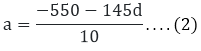Now subtracting (1) from (2),0 = -550 - 145d + 150 + 45d

0 = - 400 - 100d

100d = - 400

d = - 4

Substituting the value of d in (1)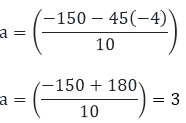So, the A.P. is 3, -1, – 5, – 9,. . .  with a = 3, d = – 4

Question: 30

In an A.P. , the first term is 2, the last term is 29 and the sum of the terms is 155, find the common difference of the A.P.

Solution:

In the given problem, we have the first and the last term of an A.P. along with the sum of all the terms of A.P. Here, we need to find the common difference of the A.P.

Here, The first term of the A.P (a) = 2

The last term of the AP (l) = 29

Sum of all the terms (Sn) = 155

Let the common difference of the A.P. be d.

So, let us first find the number of the terms (n) using the formula,

155 = n/2(2 + 29)

155(2) = n(31)

31n = 310

n = 10

Now, to find the common difference of the A.P. we use the following formula,

l = a + (n - 1)d

We get,

29 = 2 + (10 - 1)d

29 = 2 + (9)d

29 - 2 = 9d

9d = 27

d = 3

Therefore, the common difference of the A.P. is d = 3

Question: 31

Find the number of terms of the A.P. –12, –9, –6, . . . , 21. If 1 is added to each term of this A.P., then find the sum of all terms of the A.P. thus obtained.

Solution:

First term, a1 = -12

Common difference,

d = a2 - a1 = – 9 – (- 12)

= - 9 + 12 = 3

nth term = an = a + (n - 1)d

⟹ 21 = -12 + (n - 1)3

⟹ 21 = -12 + 3n - 3

⟹ 21 = 3n - 15

⟹ 36 = 3n

⟹  n = 12

Therefore, the number of terms is 12 Now, when 1 is added top each of the 12 terms, the sum will increase by 12.

So, the sum of all the terms of the A.P. thus obtained

⟹ S12 + 12 = 12/2[a + l] + 12

= 6[-12 + 21] + 12

= 6 × 9 + 12

= 66

Therefore, the sum after adding 1 to each of the term we get 66

Question: 32

The sum of first n terms of an A.P. is 3n2 + 6n. Find the nth term of this A.P.

Solution:

In the given problem, let us take the first term as a and the common difference as d. we know that nth term is given by:

an = Sn  - Sn-1

we have given here

Sn = 3n2 + 6n

So, using this to find the nth term,

⟹ an = [3n2 + 6n] - [3(n - 1)2 + 6 (n - 1)]

= [3n2 + 6n] - [3(n2 + 12 - 6n) + 6n - 6]

= 3n2 + 6n - 3n2 - 3 + 6n - 6n + 6 = 6n + 3

Therefore, the nth term of this A.P. is 6n + 3

Question: 33

The sum of n terms of an A.P. is 5n - n2. Find the nth term of this A.P.

Solution:

Let a be the first term and d be the common difference. We know that, sum of first n terms is :

Sn = n/2(2a + (n - 1)d)

It is given that sum of the first n terms of an A.P. is 5n - n2.

First term = a = S1= 5 (1) - (1)2 = 4.

Sum of first two terms = S2 = 5(2) - (2)2 = 6.

Second term = S2 - S1 = 6 - 4 = 2.

Common difference = d = Second term - First term = 2 - 4 = – 2

Also, nth term = an = a + (n - 1) d

⟹ an = 4 + (n - 1)(-2)

⟹ an = 4 - 2n + 2

⟹ an = 6 - 2n

Thus, nth term of this A.P. is 6 - 2n.

Question: 34

The sum of first n terms of an A.P. is 3n2+ 4n. Find the 25th term of this A.P.

Solution:

In the given problem, we have sum of n terms as Sn = 5n2 + 3n we know,

an = Sn - Sn-1

­We have to find out 25th term, so n = 25

⟹ a25 = S25 - S24

= [3(25)2 + 4 (25)] - [3 (24)2 + 4(24)]

= (3 × 625 + 100) - (3 × 576 + 96)

= 1975 - 1824 = 151

Therefore, its 25th term is 151

Question: 35

The sum of first n terms of an A.P. is 5n2 + 3n. If its mth term is 168, find the value of m. Also find the 20th term of this A.P.

Solution:

Here, we are given the Sum of the A.P. as

Sn = 5n2 + 3n. and its mth term is am­ = 168

Let us assume its first term as a, and the common difference as d

We know, an = Sn - Sn-1 So, here

⟹ an = (5n2 + 3n) - [5(n - 1)2 + 3 (n - 1)]

= 5n2 + 3n - [5(n2 + 1 - 2n) + 3n - 3]

= 5n2 + 3n - 5n2 -5 + 10n - 3n + 3

= 10n - 2

We are given, am = 168 Putting m in place of n, we get

⟹ am = 10m - 2

⟹ 168 = 10m - 2

⟹ 10m = 170

⟹ m = 17 and a20 = S20 - S19

= [5(20)2 + 3 (20)] - [5 (19)2 + 3(19)]

= [2000 + 60] - [1805 + 57]

= 2060 - 1862 = 198

Therefore, in the given A.P. m = 17 and the 20th term is a20  = 198

Question: 36

If the sum of first n terms of an A.P. is 4n - n2, what is the first term? What is the sum of first two terms? What is the second term? Similarly find the third, the tenth and the n­th term.

Solution:

In the given problem, the sum of n terms of an A.P. is given by the expression,

S„ = 4n - n2

So here, we can find the first term by substituting n = 1, S„ = 4n - n2 = 4(1) – 12 = 4 - 1 = 3

Similarly, the sum of first two terms can be given by, S2 = 4(2) - (2)2 = 8 - 4 = 4

Now, as we know, an = Sn - Sn-1 ­So, a2 = S2 - S1 = 4 - 3 = 1

Now, using the same method we have to find the third, tenth and nth term of the A.P.

So, for the third term,

a3 = S3 - S2 = [4(3) - (3)2] - [4(2) - (2)2] = (12 - 9) - (8 - 4) = 3 - 4 = -1

Also, for the tenth term. a10   = S10 - S9

= [44(10) - (10)2]  - [4(9) - (9)2]

= (40 - 100) - (36 - 81)

= - 60 + 45 = -15

So, for the nth term,

an = Sn - S­n-1

= [4(n) - (12)2] - [4(n - 1) - (n - 1)2]

= (4n -  n2) - (4n - 4 - n2 - 1 + 2n)

= 4n - n2 - 4n + 4 + n2 + 1 - 2n = 5 - 2n

Therefore, a = 3, S2 = 4, a2 = 1, a3 = -1, a10 = -15

Question: 37

If the sum of first n terms of an A.P. is 1/2(3n2 + 7n) , then find its nth term. Hence write the 20th term.

Solution:

Let a be the first term and d be the common difference. We know that, sum of first n terms is:

Sn = n/2(2a + (n − 1)d)

It is given that the sum of the first n terms of an A.P. is:

1/2(3n2 + 7n)

Therefore, first term (a)

= S1 = 1/2(3(1)2 + 7(1))

= 1/2(3X1 + 7)

= 1/2(10) = 5

Sum of first two terms

= S2 = 1/2(3(2)2 + 7(2))

= 1/2(3X4 + 14)

= 1/2(26) = 13

Therefore, second term = S2 - S1 = 13 - 5 = 8

Common difference = d = second term - first term = 8 - 5 = 3

Also, nth term of the A.P. is : a + (n - 1)d

= 5 + (n - 1)3 = 5 + 3n - 3 = 3n + 2

Thus, nth term of this A.P. is 3n + 2.

Now, we have to find the 20th term, so

Putting n = 2o in the above equation, we get a20 = 3 (20) + 2 = 60 + 2 = 62

Thus, 20th term of this A.P. is 62.

Question: 38

In an A.P. the sum of first n terms isFind its 25th term.

Solution:

Here the sum of first n terms is given by the expression,We need to find the 25th term of the A.P. So, we know that the nth term of an A.P. is given by, an = Sn - Sn-1  So,

a25 = S25 - S24  .... (1)

So, using the expression for the sum of n terms, we find the sum of 25 terms (S25) and the sum of 24 terms (S25), we get,Now, using the above values in (1), a21 = S25 - S24 = 1100 - 1020 = 80

Therefore, a25 = 80

Question: 39

Find the sum of all natural numbers between 1 and 100, which are divisible by 3.

Solution:

In this problem, we need to find the sum of all the multiples of 3 lying between 1 and 100.

So, we know that the first multiple of 3 after 1 is 3 and the last multiple of 3 before 100 is 99.

Also, all these terms will form an A.P. with the common difference of 3.

So here, First term (a) = 3 Last term (l) = 99

Common difference (d) = 3

So, here the first step is to find the total number of terms.

Let us take the number of terms as n.

Now, as we know, an = a + (n - 1)d

So, for the last term, 99 = 3 + (n - 1)3

⟹ 99 = 3 + 3n - 3

⟹ 99 = 3n

Further simplifying, ⟹ n = 33

Now, using the formula for the sum of n terms, i.e.

Sn = n/2[2a + (n − 1)d] we get,

⟹ S33 = 33/2[2(3) + (33 − 1)3]

= S33 = 33/2[6 + (32)3]

= S33 = 33/2[6 + 96]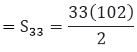= 33 (51) = 1683

Therefore, the sum of all the multiples of 3 lying between 1 and 100 is Sn = 1683

Question: 40

Find the sum of all odd numbers between (i) 0 and 50 (ii) 100 and 200.

Solution:

(i) In this problem, we need to find the sum of all odd numbers lying between 0 and 50.

So, we know that the first odd number after 0 is 1 and the last odd number before 50 is 49.

Also, all these terms will form an AP. with the common difference of 2.

So here, First term (a) = 1

Last term (0 = 49 Common difference (d) = 2

So, here the first step is to find the total number of terms.

Let us take the number of terms as n.

Now, as we know, an = a + (n -1)d

So, for the last term,

⟹ 49 = 1 + (n - 1)d

⟹ 49 = 1 + 2n - 2

⟹ 49 = 2n - 2

⟹ 49 + 1 = 2n

Further simplifying,

⟹ 50 = 2n

⟹ n = 25

Now, using the formula for the sum of n terms,

⟹ Sn = n/2[2a + (n − 1)d] for n = 25, we get

⟹ S25 = 25/2[2(1) + (25 − 1)2]

= 25/2[2 + 24 × 2]

= 25 × 25 = 625

Therefore, the sum of all the odd numbers lying between 0 and 50 is 625.

(ii) In this problem, we need to find the sum of all odd numbers lying between 100 and 200. So, we know that the first odd number after 0 is 101 and the last odd number before 200 is 199. Also, all these terms will form an AR. with the common difference of 2. So here, First term (a) = 101 Last term (an) = 199 Common difference (d) = 2 So, here the first step is to find the total number of term. Let us take the number of terms as n. Now, as we know, an = a + (n - 1)d So, for the last term,

⟹ 199 = 101 + (n - 1)2

⟹ 199 = 101 + 2n - 2

⟹ 199 = 99 + 2n

⟹ 199 - 99 = 2n

Further simplifying,

⟹ 100 = 2n

⟹ n = 50

Now, using the formula for the sum of n terms,

Sn = n/2[2(a) + (n − 1)d]

For n = 50, we get

⟹ S50 = 50/2[2(101) + (50 − 1)2]

= 25[202 + (49)2]

= 25(202 + 98) = 25

(300) = 7500

Therefore, the sum of all the odd numbers lying between 100 and 200 is 7500

Question: 41

Find the sum of all integers between 84 and 719, which are multiples of 5.

Solution:

In this problem, we need to find the sum of all the multiples of 5 lying between 84 and 719.

So, we know that the first multiple of 5 after 84 is 85 and the last multiple of 5 before 719 is 715.

Also, all these terms will form an A.P. with the common difference of 5. So here, First term (a) = 85

Last term (l) = 715 Common difference (d) = 5

So, here the first step is to find the total number of terms.

Let us take the number of terms as n.

Now, as we know, a = a +(n -1)d

So, for the last term.

715 = 85 + (n - 1)5

715 = 85 + 5n - 5

715 = 80 + 5n

715 - 80 = 5n

Further simplifying,

635 = 5n

n = 127

Now, using the formula for the sum of n terms,

Sn = n/2[2a + (n − 1)d]

For n = 127,

S127 = 127/2[2(85) + (127 − 1)5]

= 127/2[170 + 630]Therefore, the sum of all the multiples of 5 lying between 84 and 719 is 50800.

Question: 42

Find the sum of all integer between 50 and 500, which are divisible by 7.

Solution:

In this problem, we need to find the sum of all the multiples of 7 lying between 50 and 500. So, we know that the first multiple of 7 after 50 is 56 and the last multiple of 7 before 500 is 497.

Also, all these terms will form an A.P. with the common difference of 7.

So here, First term (a) = 56 Last term (l) = 497

Common difference (d) = 7 So, here the first step is to find the total number of terms.

Let us take the number of terms as n.

Now, as we know, an = a + (n - 1)d So, for the last term. 497 = 56 + (n - 1)7

⟹ 497 = 56 + 7n - 7

⟹ 497 = 49 + 7n

⟹ 497 - 49 = 7n

Further simplifying,

448 = 7n

n = 64

Now, using the formula for the sum of n terms,

Sn = n/2[2a + (n − 1)d] for n = 64, we get

S64 = 64/2[2(56) + (64 − 1)7]

= 32[112 + (63)7]

= 32[112 + 441]

= 32(553) = 17696

Therefore, the sum of all the multiples of 7 lying between 50 and 500 is 17696

Question: 43

Find the sum of all even integers between 101 and 999.

Solution:

In this problem, we need to find the sum of all the even numbers lying between 101 and 999.

So, we know that the first even number after 101 is 102 and the last even number before 999 is 998.

Also, all these terms will form an A.P. with the common difference of 2.

So here, First term (a) = 102 Last term (l) = 998

Common difference (d) = 2 So, here the first step is to find the total number of terms. Let us take the number of terms as n.

Now, as we know, an = a + (n - 1)d

So, for the last term,

⟹ 998 = 102 + (n - 1)2

⟹ 998 = 102 + 2n - 2

⟹ 998 = 100 + 2n

⟹ 998 - 100 = 2n

Further simplifying,

⟹ 898 = 2n

⟹ n = 449

Now, using the formula for the sum of n terms,

Sn = n/2[2a + (n − 1)d] For n = 449, we get

S449 = 449/2[2(102) + (449 − 1)2]

= 449/2[204 + (448)2]

= 449/2[204 + 896]

= 449/2 = 449

(550) = 246950

Therefore, the sum of all even numbers lying between 101 and 999 is 246950

Question: 44

Find the sum of all integers between 100 and 550, which are divisible by 9.

Solution:

In this problem, we need to find the sum of all the multiples of 9 lying between 100 and 550.

So, we know that the first multiple of 9 after 100 is 108 and the last multiple of 9 before 550 is 549.

Also, all these terms will form an A.P. with the common difference of 9.

So here, First term (a) = 108

Last term (l) = 549

Common difference (d) = 9 So, here the first step is to find the total number of terms.

Let us take the number of terms as n.

Now, as we know, an = a + (n - 1)d

So, for the last term.

⟹ 549 = 108 + (n - 1)d

⟹ 549 = 108 + 9n - 9

⟹ 549 = 99 + 9n

⟹ 549 - 99 = 9n

Further simplifying

⟹ 9n = 450

⟹ n = 50

Now, using the formula for the sum of n terms,

Sn = n/2[2a + (n − 1)d] We get,

Sn = 50/2[2(108) + (50 − 1)9] = 25

[216 + (49)9] = 25

(216 + 441) = 25

(657) = 16425

Therefore, the sum of all the multiples of 9 lying between 100 and 550 is 16425

Question: 45

Let there be an A.P. with first term ‘a’, common difference ‘d’. If an denotes its nth term and Sn the sum of first n terms, find.

(i) n and Sn, if a = 5 , d = 3 , and an = 50.

(ii) n and a, if an = 4 ,  d = 2 and Sn = -14.

(iii) d, if a = 3, n = 8 and Sn = 192.

(iv) a, if an = 28, Sn = 144 and n = 9.

(v) n and d, if a = 8, an = 62 and S­n­ ­= 120.

(vi) n and an , if a = 2, d = 8 and Sn = 90.

Solution:

(i) Here, we have an A.P. whose nth term (an), first term (a) and common difference (d) are given.

We need to find the number of terms (n) and the sum of first n terms (Sn).

Here, First term (a) = 5 Last term (an) = 50

Common difference (d) = 3

So here we will find the value of n using the formula, an = a + (n - 1)d

So, substituting the values in the above mentioned formula

⟹ 50 = 5 + (n - 1)3

⟹ 50 = 5 + 3n - 3

⟹ 50 = 2 + 3n

⟹ 3n = 50 - 2

Further simplifying for n, 3n = 48 n =16

Now, here we can find the sum of the n terms of the given A.P., using the formula,

Sn = (n/2)(a + l)

Where, a = the first term l = the last term

So, for the given A.P, on substituting the values in the formula for the sum of n terms of an A.P., we get,

S16 = (16/2)(5 + 50) = 8

(55) = 440

Therefore, for the given A.P. we have, n = 16 and S16 = 440

(ii) Here, we have an A.P. whose nth term (an), sum of first n terms (S0) and common difference (d) are given.

We need to find the number of terms (n) and the first term (a).

Here, Last term (l) = 4

Common difference (d) = 2

Sum of n terms (Sn) = -14

So here we will find the value of n using the formula, an = a + (n - 1)d

So, substituting the values in the above mentioned formula

⟹ 4 = a + (n - 1)2

⟹ 4 = a + 2n - 2

⟹ 4 + 2 = a + 2n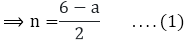Now, here the sum of the n terms is given by the formula,

S/n = (n/2)(a + l)

Where, a = the first term l = the last term So, for the given A.P, on substituting the values in the formula for the sum of n terms of an A.P., we get,

⟹ −14 = n/2(a + 4)

⟹ 14(2) = n(a + 4)

⟹ n = −28a + 4 .... (2)

Equating (1) and (2), we get,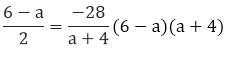= – 28(2) 6a - a2 + 24 - 4a

= – 56 - a2 + 2a + 24 + 56 = 0

So, we get the following quadratic equation,

- a 2 + 2a + 80 = 0

a2 - 2a - 80 = 0

Further solving it for a by splitting the middle term,

a2 - 2a - 80 = 0

a2 - 10a + 8a - 80 = 0

a(a - 10) + 8(a - 10) = 0

(a - 10)(a + 8) = 0

So, we get,

a - 10 = 0

a = 10 or, a + 8 = 10

a = – 8

Substituting, a = 10 in (1)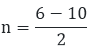n = (-4)/2

n = -2

Here, we get n as negative, which is not possible. So, we take a = - 8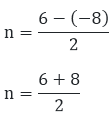n = 14/2

n = 7

Therefore, for the given A.P. n = 7 and a = – 8

(iii) Here, we have an A.P. whose first term (a), sum of first n terms (S0) and the number of terms (n) are given. We need to find common difference (d).

Here, First term (a) = 3 Sum of n terms (Sn) = 192

Number of terms (n) = 8

So here we will find the value of n using the formula, an = a + (n -1) d

So, to find the common difference of this A.P., we use the following formula for the sum of n terms of an A.P

Sn = (n/2)[2a + (n − 1)d]

Where; a = first term for the given A.P.

d = common difference of the given A.P.

n = number of terms So, using the formula for n = 8, we get,

S8 = (8/2)[2(3) + (8 − 1)d]

192 = 4[6 + 7d]

192 = 24 + 28d

28d = 192 - 24

28d = 168

d = 6

Therefore, the common difference of the given A.P. is d = 6

(iv) Here, we have an A.P. whose nth term (an), sum of first n terms (Sn) and the number of terms (n) are given. We need to find first term (a).

Here, Last term (a9) = 28

Sum of n terms (Sn) = 144

Number of terms (n) = 9 Now, a9 = a + 8d

28 = a + 8d .... (1)

Also, using the following formula for the sum of n terms of an AP

Sn = (n/2)[2a + (n − 1)d]

Where; a = first term for the given A.P.

d = common difference of the given A.P.

n = number of terms So, using the formula for n = 9, we get,

S9 = (9/2)[2a + (9 − 1)d]

144(2) = 9[2a + 8d]

288 = 18a + 72d  .... (2)

Multiplying (1) by 9, we get 9a + 72d = 252 ....  (3)

Further, subtracting (3) from (2), we get 9 a = 36 a = 4

Therefore, the first term of the given A.P. is a = 4

(v) Here, we have an A.P. whose nth term (an), sum of first n terms (Sn) and first term (a) are given.

We need to find the number of terms (n) and the common difference (d).

Here, First term (a) = 8

Last term (an ) = 62 Sum of n terms (Sn) = 210

Now, here the sum of the n terms is given by the formula,

Sn = (n/2)(a + l)

Where, a = the first term l = the last term So, for the given A.P, on substituting the values in the formula for the sum of n terms of an A.P., we get,

210 = n/2[8 + 62]

210(2) = n (70)

n = 420/70

n = 7

Also, here we will find the value of d using the formula,

an = a + (n - 1) d

So, substituting the values in the above mentioned formula

62 = 8 + (6 - 1)d

5d = 54

d = 545

Therefore, for the given A.P. n = 6 and d = 545

(vi) Here, we have an A.P. whose first term (a), common difference (d) and sum of first n terms are given.

We need to find the number of terms (n) and the nth term (an).

Here, First term (a) = 2

Sum of first nth terms (Sn) = 90

Common difference (d) = 8 So, to find the number of terms (n) of this A.P., we use the following formula for the sum of n terms of an A.P

Sn = (n/2)[2a + (n − 1)d]

Where; a = first term for the given A.P.

d = common difference of the given A.P.

n = number of terms So, using the formula for

d = 8, we get,

Sn = (n/2)[2(2) + (n – 1)8]

90 = (n/2)[4 + 8n - 8]

90(2) = n[8n - 4]

180 = 8n2 - 4n

Further solving the above quadratic equation,

8n2 - 4n - 180 = 0

2n2 - n - 45 = 0

Further solving for n, 2n2 - 10n + 9n - 45 = 0

2n(n - 5) + 9(n - 5) = 0

(2n - 9)(n - 5) = 0

Now, 2n + 9 = 0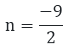Also,

n - 5 = 0

n = 5

Since, n cannot be a fraction.

Thus, n = 5 Also, we will find the value of nth term (an), using the formula,

an = a + (n - 1) d

So, substituting the values in the above formula,

an = 2 + (5 - 1) 8

an = 2 + 4 (8)

an = 2 + 32

an = 34

Therefore, for the given A.P., n = 5 and an = 34
```### Course Features

• 728 Video Lectures
• Revision Notes
• Previous Year Papers
• Mind Map
• Study Planner
• NCERT Solutions
• Discussion Forum
• Test paper with Video Solution